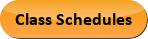Aug 14, 2022
2022-2023 College Catalog
 Select a Catalog 2022-2023 College Catalog 2021-2022 College Catalog [ARCHIVED CATALOG] 2020-2021 College Catalog [ARCHIVED CATALOG] 2019-2020 College Catalog [ARCHIVED CATALOG]
 HELP 2022-2023 College Catalog Print-Friendly Page (opens a new window)

# ENG 230 - Mechanics of Materials

3 Credits, 3 Contact Hours
3 lecture periods 0 lab periods

Introduction to the analysis and design of the mechanical properties of materials. Includes the concept of stress and strain, axially loaded members, torsion, stresses and strains in beams, analysis of stress and strain, deflections of beams, statically indeterminate beams, and columns.

Prerequisite(s): ENG 210  with a grade of B or better. Students receiving a grade of C in ENG 210  will be required to register for the ENG 230RC  course concurrently; for students receiving a grade of B or higher in ENG 210 , the ENG 230RC  course is optional.Course Learning Outcomes
1. Demonstrate the ability to compute the normal stresses and strains of prismatic bars subjected to axial loads.
2. Demonstrate the ability to compute the stress and displacement of prismatic bards subjected to temperature change, misfits, and pre-strains.
3. Demonstrate the ability to compute the stresses and strains in statically determinate and indeterminate structures.
4. Demonstrate the ability to compute the shear stresses and strains of prismatic and non-uniform shafts due to torsional loading.
5. Demonstrate the ability to compute the stresses and strains for pure and non-uniform bending.
6. Demonstrate the ability to evaluate the deflection of transverse loaded beans by integration and by method of superposition.
7. Demonstrate the ability to analyze the statically indeterminate beams by integration and by method of superposition.
8. Demonstrate the ability to compute the critical loads of columns with pinned supports and other support conditions.

Performance Objectives:
1. Apply science and engineering principles to understand the mechanical properties of materials.
2. Interpret stress-strain diagrams for typical structural materials.
3. Select an appropriate material for a given application by the comparison of stress-strain diagrams.
4. Demonstrate an understanding of fundamental concepts of Mechanics of Materials: stress and strain.
5. Compute the normal stress and strain of prismatic bars subjected to axial loads.
6. Compute the shear and bearing stresses of structural elements.
7. Compute the normal stress and strain of non-uniform bars subjected to axial loads.
8. Compute the normal stresses in statically indeterminate structures.
9. Apply equilibrium, compatibility, and force-deformation relationships to axially loaded members.
10. Compute the stress and displacement of prismatic bars subjected to temperature change.
11. Compute the stress and strain of prismatic bars subjected to misfits and prestrains.
12. Evaluate stresses on inclined sections.
13. Determine stress concentration factors.
14. Compute the angle of twist due torsion in circular shafts.
15. Compute the shear stresses of solid or hollow shafts due to torsional loading.
16. Compute the shear stresses of non-uniform shafts due to torsional loading.
17. Compute the shear stresses in statically indeterminate torsional members.
18. Apply equilibrium, compatibility, and force-deformation relationships to torsional members.
19. Design the circular shafts by analysing transmission power.
20. Construct shear forces and bending moment diagrams for various types of loaded beams.
21. Compute the moment of inertia or second moment of area for complex cross sections.
22. Compute the stress and strain for pure bending.
23. Compute the shear stress for non-uniform bending.
24. Design of beams for bending stresses.
25. Compute principle stresses, maximum shear stresses and stresses in a specified direction.
26. Utilize Mohr’s circle to compute principle stresses, maximum shear stresses and stresses in a specified direction.
27. Evaluate the deflection of transverse loaded beams by integration and by method of superposition.
28. Analyse statically indeterminate beams by integration and by method of superposition.
29. Compute the critical loads of columns with pinned supports.
30. Compute the critical loads of columns with other support conditions.
32. Apply the appropriate units of measurement to Mechanics of Materials problems and check the dimensional integrity of their solutions.

Outline:
1. Introduction to the Concept of Stress and Strain
1. Introduction to mechanics of materials
2. Forces and stresses
3. Mechanical properties of materials
4. Stress-strain diagram
5. Hooke’s Law and Poisson’s Radio; modulus of elasticity
7. Shear stress and strain
8. Bearing stress
9. Allowable stresses and allowable loads: factor of safety
1. Introduction
2. Changes in lengths of axially loaded members
3. Changes in lengths under non-uniform conditions
4. Statically indeterminate structures
5. Thermal effects
6. Misfits and prestrains
7. Stresses on inclined sections
3. Torsion
1. Introduction
2. Torsional deformations of a circular bar
3. Circular bars of linearly elastic materials
4. Non-uniform torsion
5. Stresses and strains in pure shear
6. Relationship between moduli of elasticity E and G
7. Transmission of power by circular shifts
8. Statically indeterminate torsional members
4. Stresses and Strains in Beams
1. Introduction
2. Types of beams, loads, and reactions
3. Shear-force and bending-moment diagrams
4. Review of centroids and moments of inertia of plane areas
5. Pure bending and non-uniform bending
6. Curvature of a beam
7. Longitudinal strains in beams
8. Normal stresses in beams (linearly elastic materials)
9. Design of beams of bending stresses
10. Shear stresses in beams of rectangular cross section
11. Shear stresses in beams of circular cross section
12. Shear stresses in the webs of beams with flanges
5. Analysis of Stress and Strain
1. Introduction
2. Plane stress
3. Principal stresses and maximum shear stresses
4. Mohr’s circle for plane stress
5. Triaxial stress
6. Deflections of Beams
1. Introduction
2. Deflections by integration of the bending-moment equation
3. Deflections by integration of the shear-force and load equations
4. Method of superposition
5. Moment-area method
7. Statically Indeterminate Beams
1. Introduction
2. Types of statically indeterminate beams
3. Analysis by the differential equations of the deflection curve
4. Method of superposition
8. Columns
1. Introduction
2. Buckling and stability
3. Columns with pinned ends
4. Columns with other support conditions
5. Columns with eccentric axial loads
6. Elastic and inelastic: column behavior/inelastic buckling
7. Design formulas for columns

Effective Term: